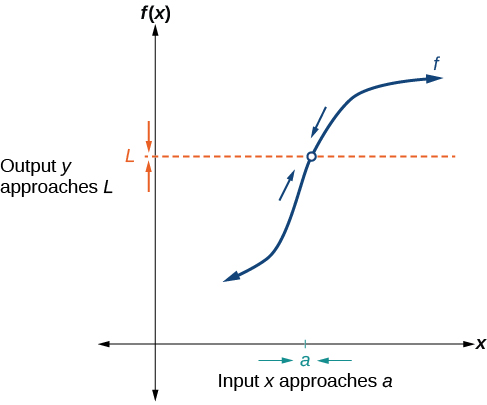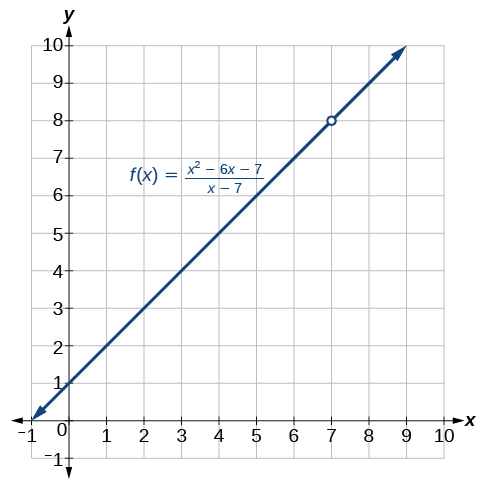12.1 Finding limits: numerical and graphical approaches

 Page 1 / 10
In this section, you will:
• Understand limit notation.
• Find a limit using a graph.
• Find a limit using a table.

Intuitively, we know what a limit    is. A car can go only so fast and no faster. A trash can might hold 33 gallons and no more. It is natural for measured amounts to have limits. What, for instance, is the limit to the height of a woman? The tallest woman on record was Jinlian Zeng from China, who was 8 ft 1 in. https://en.wikipedia.org/wiki/Human_height and http://en.wikipedia.org/wiki/List_of_tallest_people Is this the limit of the height to which women can grow? Perhaps not, but there is likely a limit that we might describe in inches if we were able to determine what it was.

To put it mathematically, the function whose input is a woman and whose output is a measured height in inches has a limit. In this section, we will examine numerical and graphical approaches to identifying limits.

Understanding limit notation

We have seen how a sequence    can have a limit, a value that the sequence of terms moves toward as the nu mber of terms increases. For example, the terms of the sequence

$1,\frac{1}{2},\frac{1}{4},\frac{1}{8}...$

gets closer and closer to 0. A sequence is one type of function, but functions that are not sequences can also have limits. We can describe the behavior of the function as the input values get close to a specific value. If the limit of a function $\text{\hspace{0.17em}}f\left(x\right)=L\text{,}\text{\hspace{0.17em}}$ then as the input $\text{\hspace{0.17em}}x\text{\hspace{0.17em}}$ gets closer and closer to $\text{\hspace{0.17em}}a,$ the output y -coordinate gets closer and closer to $\text{\hspace{0.17em}}L.\text{\hspace{0.17em}}$ We say that the output “approaches” $\text{\hspace{0.17em}}L.$

[link] provides a visual representation of the mathematical concept of limit. As the input value $\text{\hspace{0.17em}}x\text{\hspace{0.17em}}$ approaches $\text{\hspace{0.17em}}a,$ the output value $\text{\hspace{0.17em}}f\left(x\right)\text{\hspace{0.17em}}$ approaches $\text{\hspace{0.17em}}L.$The output ( y --coordinate) approaches   L   as the input ( x -coordinate) approaches   a .

We write the equation of a limit as

$\underset{x\to a}{\mathrm{lim}}f\left(x\right)=L.$

This notation indicates that as $\text{\hspace{0.17em}}x\text{\hspace{0.17em}}$ approaches $\text{\hspace{0.17em}}a\text{\hspace{0.17em}}$ both from the left of $\text{\hspace{0.17em}}x=a\text{\hspace{0.17em}}$ and the right of $\text{\hspace{0.17em}}x=a,$ the output value approaches $\text{\hspace{0.17em}}L.$

Consider the function

$f\left(x\right)=\frac{{x}^{2}-6x-7}{x-7}.$

We can factor the function as shown.

Notice that $\text{\hspace{0.17em}}x\text{\hspace{0.17em}}$ cannot be 7, or we would be dividing by 0, so 7 is not in the domain of the original function. In order to avoid changing the function when we simplify, we set the same condition, $\text{\hspace{0.17em}}x\ne 7,$ for the simplified function. We can represent the function graphically as shown in [link] .Because 7 is not allowed as an input, there is no point at   x = 7.

What happens at $\text{\hspace{0.17em}}x=7\text{\hspace{0.17em}}$ is completely different from what happens at points close to $\text{\hspace{0.17em}}x=7\text{\hspace{0.17em}}$ on either side. The notation

$\underset{x\to \text{\hspace{0.17em}}7}{\mathrm{lim}}f\left(x\right)=8$

indicates that as the input $\text{\hspace{0.17em}}x\text{\hspace{0.17em}}$ approaches 7 from either the left or the right, the output approaches 8. The output can get as close to 8 as we like if the input is sufficiently near 7.

What happens at $\text{\hspace{0.17em}}x=7?\text{\hspace{0.17em}}$ When $\text{\hspace{0.17em}}x=7,$ there is no corresponding output. We write this as

This notation indicates that 7 is not in the domain of the function. We had already indicated this when we wrote the function as

Notice that the limit of a function can exist even when $\text{\hspace{0.17em}}f\left(x\right)\text{\hspace{0.17em}}$ is not defined at $\text{\hspace{0.17em}}x=a.\text{\hspace{0.17em}}$ Much of our subsequent work will be determining limits of functions as $\text{\hspace{0.17em}}x\text{\hspace{0.17em}}$ nears $\text{\hspace{0.17em}}a,$ even though the output at $\text{\hspace{0.17em}}x=a\text{\hspace{0.17em}}$ does not exist.

find the equation of the line if m=3, and b=-2
graph the following linear equation using intercepts method. 2x+y=4
Ashley
how
Wargod
what?
John
ok, one moment
UriEl
how do I post your graph for you?
UriEl
it won't let me send an image?
UriEl
also for the first one... y=mx+b so.... y=3x-2
UriEl
y=mx+b you were already given the 'm' and 'b'. so.. y=3x-2
Tommy
"7"has an open circle and "10"has a filled in circle who can I have a set builder notation
x=-b+_Гb2-(4ac) ______________ 2a
I've run into this: x = r*cos(angle1 + angle2) Which expands to: x = r(cos(angle1)*cos(angle2) - sin(angle1)*sin(angle2)) The r value confuses me here, because distributing it makes: (r*cos(angle2))(cos(angle1) - (r*sin(angle2))(sin(angle1)) How does this make sense? Why does the r distribute once
so good
abdikarin
this is an identity when 2 adding two angles within a cosine. it's called the cosine sum formula. there is also a different formula when cosine has an angle minus another angle it's called the sum and difference formulas and they are under any list of trig identities
strategies to form the general term
carlmark
How can you tell what type of parent function a graph is ?
generally by how the graph looks and understanding what the base parent functions look like and perform on a graph
William
if you have a graphed line, you can have an idea by how the directions of the line turns, i.e. negative, positive, zero
William
y=x will obviously be a straight line with a zero slope
William
y=x^2 will have a parabolic line opening to positive infinity on both sides of the y axis vice versa with y=-x^2 you'll have both ends of the parabolic line pointing downward heading to negative infinity on both sides of the y axis
William
y=x will be a straight line, but it will have a slope of one. Remember, if y=1 then x=1, so for every unit you rise you move over positively one unit. To get a straight line with a slope of 0, set y=1 or any integer.
Aaron
yes, correction on my end, I meant slope of 1 instead of slope of 0
William
what is f(x)=
I don't understand
Joe
Typically a function 'f' will take 'x' as input, and produce 'y' as output. As 'f(x)=y'. According to Google, "The range of a function is the complete set of all possible resulting values of the dependent variable (y, usually), after we have substituted the domain."
Thomas
Sorry, I don't know where the "Â"s came from. They shouldn't be there. Just ignore them. :-)
Thomas
Darius
Thanks.
Thomas
Â
Thomas
It is the Â that should not be there. It doesn't seem to show if encloses in quotation marks. "Â" or 'Â' ... Â
Thomas
Now it shows, go figure?
Thomas
what is this?
i do not understand anything
unknown
lol...it gets better
Darius
I've been struggling so much through all of this. my final is in four weeks 😭
Tiffany
this book is an excellent resource! have you guys ever looked at the online tutoring? there's one that is called "That Tutor Guy" and he goes over a lot of the concepts
Darius
thank you I have heard of him. I should check him out.
Tiffany
is there any question in particular?
Joe
I have always struggled with math. I get lost really easy, if you have any advice for that, it would help tremendously.
Tiffany
Sure, are you in high school or college?
Darius
Hi, apologies for the delayed response. I'm in college.
Tiffany
how to solve polynomial using a calculator
So a horizontal compression by factor of 1/2 is the same as a horizontal stretch by a factor of 2, right?
The center is at (3,4) a focus is at (3,-1), and the lenght of the major axis is 26
The center is at (3,4) a focus is at (3,-1) and the lenght of the major axis is 26 what will be the answer?
Rima
I done know
Joe
What kind of answer is that😑?
Rima
I had just woken up when i got this message
Joe
Can you please help me. Tomorrow is the deadline of my assignment then I don't know how to solve that
Rima
i have a question.
Abdul
how do you find the real and complex roots of a polynomial?
Abdul
@abdul with delta maybe which is b(square)-4ac=result then the 1st root -b-radical delta over 2a and the 2nd root -b+radical delta over 2a. I am not sure if this was your question but check it up
Nare
This is the actual question: Find all roots(real and complex) of the polynomial f(x)=6x^3 + x^2 - 4x + 1
Abdul
@Nare please let me know if you can solve it.
Abdul
I have a question
juweeriya
hello guys I'm new here? will you happy with me
mustapha
The average annual population increase of a pack of wolves is 25.
how do you find the period of a sine graph
Period =2π if there is a coefficient (b), just divide the coefficient by 2π to get the new period
Am
if not then how would I find it from a graph
Imani
by looking at the graph, find the distance between two consecutive maximum points (the highest points of the wave). so if the top of one wave is at point A (1,2) and the next top of the wave is at point B (6,2), then the period is 5, the difference of the x-coordinates.
Am
you could also do it with two consecutive minimum points or x-intercepts
Am
I will try that thank u
Imani
Case of Equilateral Hyperbola
ok
Zander
ok
Shella
f(x)=4x+2, find f(3)
Benetta
f(3)=4(3)+2 f(3)=14
lamoussa
14
Vedant
pre calc teacher: "Plug in Plug in...smell's good" f(x)=14
Devante
8x=40
Chris
Explain why log a x is not defined for a < 0
the sum of any two linear polynomial is whatByByBy Sam LuongBy